Surface Tension

Key Concepts

• A surface is defined as the boundary between a liquid and a gas, or, as the boundary between a solid and a gas.
• Surface tension is the tendency for a liquid to minimize its surface area.
• The three-dimensional shape that has the smallest surface area for a given volume is the sphere.
Droplets of liquid are spherical in shape.
• Surface tension is given the symbol γo.
• Units of measurement of surface tension are:
(i) force per unit length (Nm-1)
or
(ii) energy per unit area (Jm-2).
• Different liquids have different values for surface tension (γo)
The greater the intermolecular forces acting between liquid particles, the greater the surface tension of the liquid.
The attraction between particles in a pure liquid is known as cohesion, or as a cohesive force.
• Surface tension is dependent on temperature.
Hot liquids have a lower surface tension than cold liquids.

No ads = no money for us = no free stuff for you!

Defining Surface Tension

 The density of steel is about 8 g cm-3 while the density of water is about 1 g cm-3 (at room temperature and pressure). If you drop a steel needle into a cup of water, the needle will sink because the density of the steel in the needle is greater than the density of the liquid water. But, if you very gently lower the needle onto the surface of the water without breaking the surface of the water, the needle will float, even though the density of the steel is much greater than the density of the water. If you were to observe the steel needle floating on the water using a magnifying glass, you would see how the surface of the liquid water is bent around and under the needle, as shown in the diagram on the right.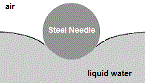This bending of the surface of the water without breaking is as a result of the intermolecular forces acting between water molecules in the liquid, and is known as surface tension.

Every particle making up a liquid, such as a water molecule in liquid water, is attracted to the other particles that surround it in the liquid. These forces of attraction between the particles are known as intermolecular forces.

The intermolecular forces of attraction between particles in a liquid are shown as arrows pointing towards the centre of a particle in the representation of a liquid shown on the right.

Consider a particle in the middle of a volume of the liquid, shown in the representation on the right as O
A particle in the middle of the liquid is completely surrounded by other particles so the forces of attraction on this particle are equal in all directions, we say the forces of attraction are balanced, and this is shown by pairs of arrows facing in opposite directions.

Now consider one of the particles on an edge, or at the surface, shown as O in the representation on the right.
A particle at the surface is not completely surrounded by other particles, so the forces of attraction acting on this particle are not equal, that is, the forces are unbalanced, as shown by a single arrow pointing toward the centre of the particle in the representation on the right.

 ↓ ↓ ↓ → O →← O →← O ← ↓ ↑ ↓ ↑ ↓ ↑
 → O →← O →← O ← ↓ ↑ ↓ ↑ ↓ ↑
 → O →← O →← O ← ↑ ↑ ↑

The unbalanced forces of attraction acting on a particle at the surface of a liquid tends to pull the particle back in towards the centre of the liquid.
This means that the forces acting between the particles at the surface of the liquid are actually stronger than those acting between particles in the middle of the volume of the liquid.
This strengthening of the intermolecular force acting on each particle at the surface results in the surface of the liquid resisting changes such as being stretched or broken. The particles at the surface of the liquid are said to be under tension, and the effect is very much like there being a thin, invisible film stretched over the surface of the liquid. It is this tension between the particles on the surface of a liquid (its surface tension) that enables a more dense object to float on top of a less dense liquid if the denser object is lowered into position very carefully.

Do you know this?

Play the game now!

Intermolecular Forces and Surface Tension

The particles making up a pure liquid are all attracted to each other by intermolecular forces.
Chemists refer to this attraction between identical particles within a liquid as cohesion.

The stronger the intermolecular forces acting between the particles in a liquid, the stronger the cohesive forces, the greater the surface tension of the liquid.

Consider the surface tension values given on the right for different liquids at 20oC.

Hexane, C6H14, has the lowest surface tension of all the liquids given here.
Hexane is a non-polar molecule, the only intermolecular forces acting between hexane molecules in the liquid will be the weakest of all the intermolecular forces, London forces (also known as dispersion forces). Since the intermolecular forces acting between the hexane molecules are so weak, the cohesive force acting between the molecules is small, and the surface tension of liquid hexane is low.

Liquid
at 20oC
Surface Tension
o / mN m-1)
mercury 485
water 72.8
acetic acid
(ethanoic acid)
27.6
ethanol
(ethyl alcohol)
22.3
hexane 18.4

Ethanol, C2H5OH, is a polar molecule. There is partial negative charge (δ-) on the oxygen atom of the OH group, and a partial positive charge (δ+) on the H atom of the OH group: C2H5Oδ-Hδ+. So, the oxygen atom of one ethanol molecule is attracted to the partially oppositely charged hydrogen atom on another ethanol molecule. This is called hydrogen bonding and it is a stronger intermolecular force of attraction than London forces (or dispersion forces). Molecules of ethanol are attracted to each other by both the weak intermolecular forces known as London forces or dispersion forces, as well as by the stronger hydrogen bonds. Compared to non-polar hexane molecules, ethanol molecules exert a stronger force of attraction on each other, the cohesive forces are greater, and so the surface tension of liquid ethanol is greater than the surface tension of liquid hexane.

Acetic acid (ethanoic acid), CH3COOH, has a greater surface tension than ethanol because, as well as all acetic acid molecules being attracted to each other by the weak intermolecular forces known as London or dispersion forces, an acetic acid molecule can form two hydrogen bonds, more hydrogen bonds means greater force of cohesion, which results in a greater surface tension.

The surface tension for water is even greater than that of acetic acid.
The water molecule is also polar, δ+H-Oδ--Hδ+, and it can form two hydrogen bonds, but, the water molecule is also very, very small compared to an acetic acid molecule, so that the intermolecular forces experienced between water molecules is much greater than that experienced between much larger acetic acid molecules.

The surface tension of mercury is enormous, almost six times greater than that of water. So the intermolecular forces acting between mercury atoms in the liquid must also be enormous, much, much, stronger than the intermolecular forces acting between hexane, ethanol, acetic acid and water.
These much stronger forces are called metallic bonds.

The stronger the intermolecular forces acting between the particles in a liquid, the stronger the cohesive forces, the greater the surface tension of the liquid.
If you wanted to float a steel needle on the surface of these liquids, as we did in the first section, then it would be easiest to float the needle on mercury which has the greatest surface tension, and hardest to float it on hexane which has the lowest surface tension.

Do you understand this?

Take the test now!

Shape of a Droplet of Liquid

The unbalanced forces of attraction acting on a particle of the liquid at the surface cause this particle to experience a net force toward the body of the liquid, that is, away from the surface. Since all the particles at the surface are experiencing the same pull towards the body of the liquid, the surface of the liquid will take a form that minimises the number of particles at the surface, or, to put it another way, to maximise the number of particles in the body of the liquid. This means that a droplet of the liquid will make a 3-dimensional shape with the lowest surface area for that volume of liquid.
The three dimensional shape that has the smallest surface area for a given volume is the sphere, so droplets of liquid form spheres.

Consider 1 mL, or 1 cm3
(1 cc), of a liquid such as water.

The table on the right compares the surface areas of different three-dimensional shapes that have a volume of 1 cm3.

As the number of sides of the 3D shape increases from 4 (tetrahedron) to 6 (cube) to 8 (octahedron), the surface area decreases from 7.21 to 6 to 5.72 cm2.
Increasing the number of sides enclosing a given volume, decreases the surface area.

3D shape volume = 1 cm3 surface area surface area
to volume ratio
sphere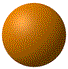volume = V = 1 cm3
V = 4/3 πr3
r = (3V/)1/3
radius = r = 0.62 cm
surface area = 4πr2
= 4π(0.62)2
= 4.83 cm2
4.83 : 1
octahedron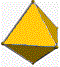volume = V = 1 cm3
V = 1/3√2l3
l = [(3V)2/2]1/3
length = l = 1.65 cm
surface area = 2√3l2
= 2√(3 x 1.652)
= 5.72 cm2
5.72 : 1
cube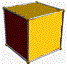volume = V = 1 cm3
V = l3
l = V1/3
length = l = 1 cm
surface area = 6l2
= 6 x 12
= 6 cm2
6 : 1
tetrahedron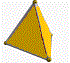volume = V = 1 cm3
V = (√2l3)/12
l = [(12V)2/2]1/3
length = l = 4.16 cm
surface area = √3l2
= √3x(4.16)2
= 7.21 cm2
7.21 : 1
This can be shown clearly if we plot a graph of the surface area to volume ratio against the number of sides for the 3D shape as shown on the right.
 Surface Area to Volume Ratios for Given Number of Sides surface area : volume Number of Sides
From the graph we can see that the line is flattening out by the time we get to 20 sides.
A sphere can then be thought of as the 3D shape in which the limit of the number of sides has been reached (approaches infinity), and its surface area to volume ratio will be the flat part of the extended line.
Therefore, a sphere is the 3D shape that has the smallest surface area to volume ratio.

Can you apply this?

Join AUS-e-TUTE!

Take the test now!

Effect of Temperature on Surface Tension

Consider the data below which gives the surface tension for liquid water at different temperatures.

temperature
oC
surface tension
mN m-1
0 75.6
20 72.8
25 72.0
50 67.9
100 58.9
 Effect of Temperature on Surface Tension of Water surface tension mN m-1 Temperature/ oC

As the temperature of the water increases from 0 to 100oC, the surface tension of the water decreases from 75.6 to 58.9 mN m-1.

When a liquid is heated, the particles making up the liquid gain energy and move faster.
This means that the energy being added to the liquid is weakening the attractive, intermolecular, forces acting between the particles in the liquid.
So, the cohesive forces in the liquid must be decreasing, which results in a decrease in the surface tension of the liquid.

Can you apply this?

Join AUS-e-TUTE!

Take the test now!